# Q Factor: What is it? (And How Do You Measure It?)

Contents

## What is Q Factor?

Q factor (also known as Quality Factor or Q-factor) is defined as a dimensionless parameter that describes the underdamped condition of an oscillator or resonator. The quality factor measures the performance of a coil, a capacitor, or an inductor in terms of its losses and resonator bandwidth.

The definition of Quality Factor was originally envisaged by an engineer called K. S. Johnson of the U.S. Department of Engineering of the Western Electric Company. He measured the efficiency and consistency of the various coils. During his studies, he developed the idea of the Q factor. Curiously, his option of letter Q was made not because of the word quality factor. He used since all the other letters of the alphabet were taken.

The Q factor implies energy losses within a resonant device that might be anything from a mechanical pendulum, an entity in a mechanical structure, or from an electrical circuit, such as a resonant circuit.

Q factor shows the energy loss due to the quantity of energy contained in the design. Thus, the larger the Q factor, the lower the rate of energy loss, and hence the slower the oscillations. They’re going to get a low degree of damping, and they’re going to ring longer.

In the case of electronic circuits, energy losses are caused by resistance inside the circuit. While this may occur somewhere within the circuit, the main trigger of resistance is inside the inductor. Although the Q factor of the element corresponds to losses, it relates directly to the bandwidth of the resonator with regard to its center frequency.

## How to Measure Q Factor?

The instrument used to calculate the quality factor of the radio frequency circuit is called the Q-meter. The Q metre calculates the quality factor of the circuit and indicates the total energy dissipated by the circuit. It also describes the characteristics of the coil and the capacitor. The Q metre is used in a laboratory to measure the radio frequency of the coils.

### Working Principle of Q meter

The Q meter operates on the principle of series resonance. Resonance is the state that occurs in the circuit when their inductance and capacitance are of equal magnitude. They cause energy that oscillates between the electrical and the magnetic fields of the capacitor and the inductor. The Q-meter works based on the characteristics of the resistance, inductance, and capacitance of the resonant series circuit.

As we discussed, every Q meter works on the principle of a series resonance. So, at resonance,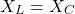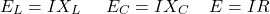where E is the applied voltage,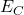is the capacitor voltage,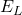is the inductor voltage,is the inductive reactance,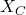is the capacitive reactance, R is the coil resistance and I is the circuit current.

Hence, Q factor is given as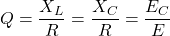From the above equation, if E is kept constant the voltage across the capacitor can be estimated by a voltmeter calibrated to read directly in terms of Q.

## Q Factor Formula

The quality factor (Q) of the resonator can be characterized as the frequency of the resonator divided by the bandwidth of the resonator.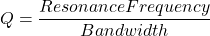where the resonance frequency and bandwidth must be given in the same units.

The Q factor formula differs for each type of circuit. Discussed below are few Q factor formula for various electrical circuits.

### Q Factor of Inductor

The quality factor of the specific reactive component depends on the frequency at which it is tested. This is usually the resonant frequency of the circuit in which it is included.

The Quality factor of a resonant circuit using that inductor that includes its series loss and a perfect capacitor is used to determine the Q factor of an inductor with a series loss resistance. The quality factor of an inductor is given as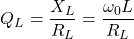where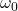is the resonance frequency in radians per second, L is the inductance,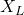is the inductive reactance, and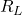is the series resistance of the inductor.

### Q Factor of Capacitor

The Q factor of a capacitor with a series loss resistance is the alike as the Q factor of a resonant circuit utilising that capacitor with a perfect inductor. The Q factor of a capacitor is given as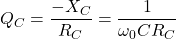whereis the resonance frequency in radians per second, C is the capacitance,is the inductive reactance, and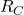is the series resistance of the capacitor.

In general, the Q factor of a resonator including a series sequence of a capacitor and an inductor can be resolved from the Q factor values of the components. This is applicable to whether their losses arise from series resistance or differently.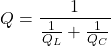### Q Factor of LC Circuits

In the parallel LC circuit, the resistance R of the inductor is in series with the inductance L. This is one of the major problems in the LC circuit. But, Q is the same as in the series circuit. Hence the Q factor is given as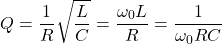where the resistance, inductance and capacitance of the tuned circuit are R, L and C.

### Q Factor of RLC Circuits

A tuned radio frequency receiver is a class of radio receiver that contains one or more tuned radio frequency amplifier stages accompanied by a demodulator circuit to collect an audio signal and typically an audio frequency amplifier.

The Quality factor for an ideal series RLC circuit and for a tuned radio frequency receiver (TRF) is given below.where the resistance, inductance and capacitance of the tuned circuit are R, L and C. When the resistance of the sequence is greater, the lower will be the value of Q in the circuit.

For a parallel RLC circuit, the Q factor will be the inverse of the series RLC circuit.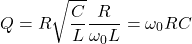Imagine a circuit where R, L and C are all parallel. The lower the parallel resistance, the more effective it would have on the damping of the circuit, and thus the lower the Q. This is useful in filtering design for bandwidth determination.

## Q Factor Transfer Function

The filter response can be represented by the s-domain transfer function. The parameter S comes is obtained from Laplace transformation and represents complex frequency filters also have a quality factor Q and it is often expressed as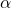.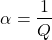This is commonly known as the damping ratio.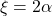.

### Q Factor Low Pass Filter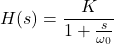This transfer function is a mathematical explanation of the frequency-domain action of the first-order low-pass filter. The same transfer function can be expressed in terms of quality factor andalso.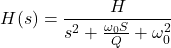where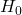is the pass band gain and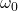is the cutoff frequency.

### Q Factor High Pass Filter

To transform a low pass filter to a high pass filter, the numerator of the low pass filter’s transfer function is changed to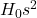. The output of the high-pass filter is similar in form to the low-pass filter but flipped in frequency.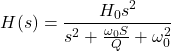### Q Factor Band Pass Filter

The numerator of the low pass filter is changed to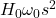, to obtain the bandpass filter function.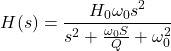whereis the cutoff frequency.is the gain of the circuit.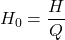In band pass filter design, Q is known as selectivity of the filter.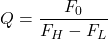where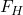and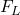are the frequencies where the response is –3 dB from the maximum

### Q Factor Notch (Bandstop) Filter

When the numerator of the bandpass filter is changed to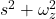, the bandstop filter can be obtained (also known as the notch filter or band-reject filter). The narrow-band-reject filter will be referred to as the notch filter and the wide-band-reject filter will be referred to as the band-reject filter.

The transfer function for a band reject filter is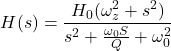## Q factor and Damping

The Q factor is used to determine the qualitative behavior of simple damped oscillators. In physical systems, damping is created by processes that dissipate the energy stored in the oscillation.

Overdamped Condition: A system is said to be overdamped when the quality factor is low (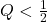). This system does not oscillate at all. So when it is shifted from its steady-state balanced output, it returns to the same position by exponential decay, reaching the steady-state value asymptotically.

It has an impulse response that is the sum of the two decaying exponential functions with varying rates of decay. A second-order low-pass filter with a very low-quality factor has almost a first-order phase response. The output of the device responds to the step input by slowly rising towards the asymptote.

Underdamped Condition: A system is said to be overdamped when the quality factor is low (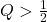). Underdamped systems integrate the oscillation at a specific frequency with the decay of the signal amplitude.

Underdamped systems with a poor quality factor may oscillate only once or a few times before dying out. If the quality component increases, the relative quantity of damping decreases. More broadly, the performance of a second-order low-pass filter with a very high-quality factor refers to the step input by rapidly increasing above, oscillating through, and gradually converging to a steady-state value.

Critically Damped Condition: A system is said to be critically damped when the quality factor is intermediate (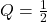). As an over-damped system, the output doesn’t really oscillate and therefore does not overshoot its steady-state output.

Like an underdamped system, the output of this system responds rapidly to a unit step input. Critical damping results in the best answer to the final value without overshooting.

### Quality factors of common systems

A good example of a critically damped system is the unit-gain Sallen-Key low-pass filter topology. It has an equal number of capacitors and resistors. So the quality factor isAn example of the underdamped condition is a second-order Butterworth filter. The quality factor is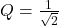## Effects of Q Factor

There are several reasons why the Q factor is significant when working with RF tuned circuits. Typically a high level of Q factor is advantageous, but some implementations may require a given level of Q factor. Some of the concerns associated with the Q factor in RF tuned circuits are summarised below.

Bandwidth:

If the quality factor increases, the bandwidth of the tuned circuit filters is decreased. As losses reduce, the tuned circuit becomes sharper as energy is best retained in the circuit. It can be shown that as the Q factor increases, the bandwidth of 3 dB decreases, and the total output of the tuned circuit increases. In certain cases, a high Q factor is necessary to ensure that the relevant degree of selectivity is achieved.

Accordingly, in many applications, the amount of Q factor needed must be calculated in order to provide the total output necessary to satisfy the criteria for broad bandwidth and the acceptable rejection of undesirable signals.

Oscillator phase noise:

Any oscillator can produce phase noise. This involves random changes in the signal process. This is manifested as a disruption that extends out from the primary carrier. It would be expected, this noise is not desired and thus needs to be reduced.

Ringing:

If the Q factor of the resonant circuit increases, the losses decrease. This ensures that any oscillation set up inside the circuit would take longer to die. In other words, the circuit appears to “ring” more. In fact, this is suitable for use in the oscillator circuit since it is simpler to set up and sustain the oscillation since less energy is wasted in the tuned circuit.

Want To Learn Faster? 🎓
Get electrical articles delivered to your inbox every week.
No credit card required—it’s 100% free.